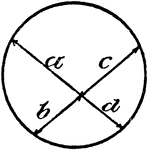### Model Of Geometric Proportions In A Circle

An illustration showing a model of a circle with intersecting chords that illustrates the following…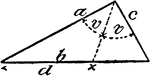### Model Of Geometric Proportions In A Triangle

An illustration showing a model of a triangle that illustrates the following relationship: a:c = d:(b…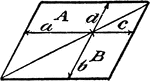### Model Of Geometric Proportions

An illustration showing a quadrilateral model that illustrates the following relationships: a:b = c:d,…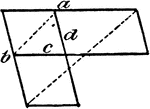### Model Of Geometric Proportions

An illustration showing a model that illustrates the following relationships: a:b = c:d, ad = bc. Product…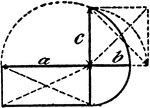### Model Of Geometric Proportions

An illustration showing a model that illustrates the following relationships: a:b = c:b, ab = c²,…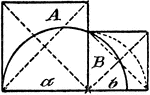### Model Of Geometric Proportions

An illustration showing a model that illustrates the following relationship: A:B = a:b.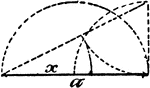### Model Of Geometric Proportions

An illustration showing a model that illustrates the following relationships: a:x = x:a - x, x = √(a²…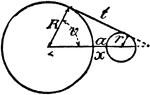### Model Of Geometric Relationships In 2 Circles

An illustration showing a model of 2 circles with tangent lines, diameters, and radii that illustrates…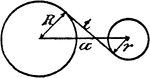### Model Of Geometric Relationships In 2 Circles

An illustration showing a model of 2 circles with tangent lines, diameters, and radii that illustrates…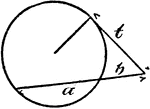### Model Of Geometric Relationships In A Circle

An illustration showing a model of a circle with an exterior angle formed between a tangent and a secant…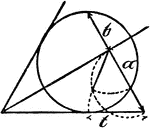### Model Of Geometric Relationships In A Circle

An illustration showing a model of a circle with angles formed between tangents and secants that illustrates…